## Given the points J(-4,0) and K(0, 4), find the coordinates of the point P on directed line segment JK that partitions segment JK in th

Question

Given the points J(-4,0) and K(0, 4), find the coordinates of the point P on directed
line segment JK that partitions segment JK in the ratio 3:1

in progress 0
6 months 2021-08-25T11:57:11+00:00 1 Answers 0 views 0

## Answers ( )

Step-by-step explanation:

We are given a ratio for this problem so we will use the formula for when we are given a ratio (as opposed to the formula for when you are given that the point you’re looking for is a fraction of the way from one point to another, like 1/3 of the way from point A to point B. That’s a different formula).

The formulas are for the x and y coordinates of the point in question: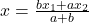and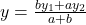where

a = 3 (from the ratio),

b = 1 (from the ratio),

x1 = -4, y1 = 0 (from point J)

x2 = 0, y2 = 4 (from point K). Filling in for x first: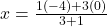gives us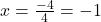and now for y: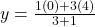gives us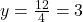Therefore, the coordinates that partition that segment into the ratio of 3:1 are (-1, 3)